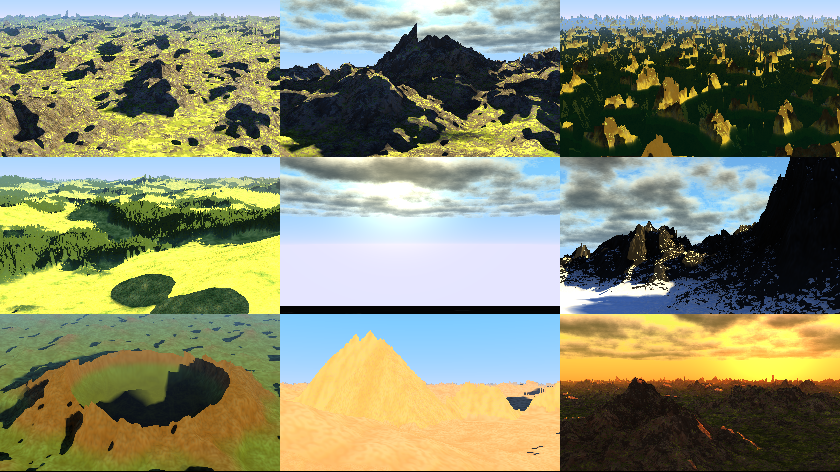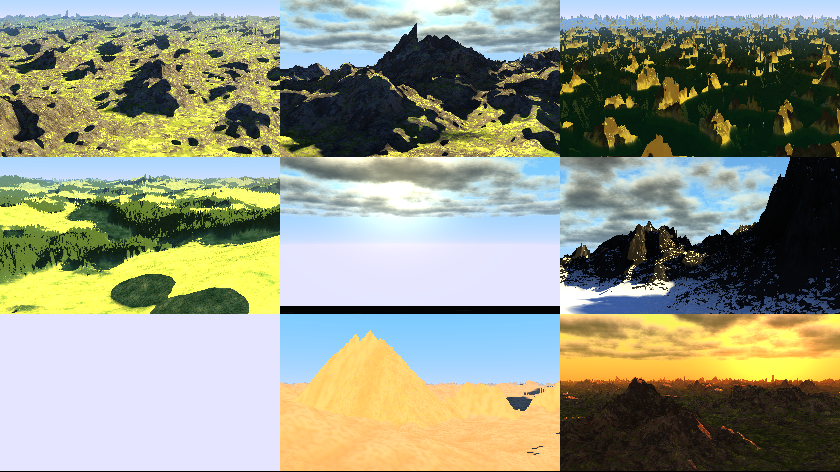## again: gen gas vs. gen gcc !

General FreeBASIC programming questions.
D.J.Peters
Posts: 8019
Joined: May 28, 2005 3:28
Contact:

### again: gen gas vs. gen gcc !

I was thinking or dreaming :-) the problem gas vs gcc was fixed in the past but isn't.
(we saw the problem with fbraytracer.bas before)

I disabled randomize(XXX) so the results of gas and gcc should not be the same but near the same.
You can see in the second image the bottom left scene isn't rendered with -gen gcc
(fbc 32-bit -gen gcc and fbc 64-bit produce the same result)

Give it a try if you like.
right click save as: gasvsgcc.bas

Joshy
fbc -gen gas gasvsgcc.basfbc -gen gcc gasvsgcc.basfrisian
Posts: 249
Joined: Oct 08, 2009 17:25

### Re: again: gen gas vs. gen gcc [FIXED]

Replace the function Crater.sphereFunc with one of the three new versions.
Change the test condition in if then.

Code: Select all

`function Crater.sphereFunc(x as REAL, z as REAL, r as REAL) as REAL  x/=r : z/=r  dim as REAL tmp =1 - x*x - z*z  if tmp > 0 then tmp = rSqr(tmp)  tmp=rMax(tmp,0)  return tmp*rend function`

Swapped two lines.

Code: Select all

`function Crater.sphereFunc(x as REAL, z as REAL, r as REAL) as REAL  x/=r : z/=r  dim as REAL tmp =1 - x*x - z*z  tmp=rMax(tmp,0)  if tmp then tmp = rSqr(tmp)  return tmp*rend function`

Rewrote the if then statement.

Code: Select all

`function Crater.sphereFunc(x as REAL, z as REAL, r as REAL) as REAL  x/=r : z/=r  dim as REAL tmp =1 - x*x - z*z  If tmp > 0 Then      Return rSqr(tmp) * r  Else      Return 0  End Ifend function`

The handling of a negative number in the square root algorithm seems to be different in FB and C, both work with negative numbers.
Negative numbers need to be handled by the programmer, they are not handled by the FB or C.